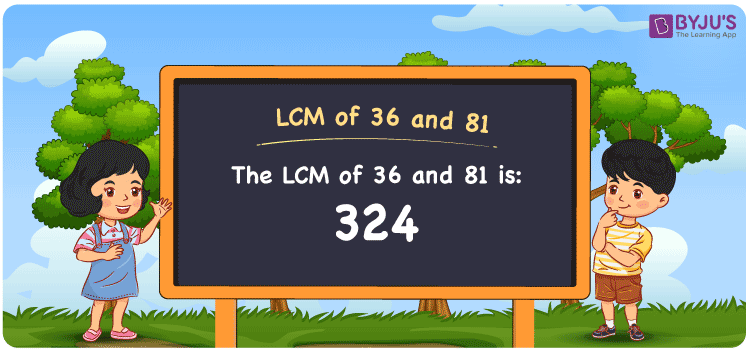# LCM of 36 and 81

LCM of 36 and 81 is 324. LCM is a very crucial topic in Mathematics and has a huge application in our day to day activities. The least common multiple of 36 and 81 is the value we get from the common multiples. (36, 72, 108, 144, 180, 216, ….) and (81, 162, 243, 324, 405, 486, 567, …..) are the first few multiples of 36 and 81. The LCM value can be found with the help of division, prime factorisation and by listing the multiples.

Also read: Least common multiple

## What is LCM of 36 and 81?

The answer to this question is 324. The LCM of 36 and 81 using various methods is shown in this article for your reference. The LCM of two non-zero integers, 36 and 81, is the smallest positive integer 324 which is divisible by both 36 and 81 with no remainder.## How to Find LCM of 36 and 81?

LCM of 36 and 81 can be found using three methods:

• Prime Factorisation
• Division method
• Listing the multiples

### LCM of 36 and 81 Using Prime Factorisation Method

The prime factorisation of 36 and 81, respectively, is given by:

36 = 2 x 2 x 3 x 3 = 2² x 3²

81 = 3 x 3 x 3 x 3 = 3⁴

LCM (36, 81) = 324

### LCM of 36 and 81 Using Division Method

We’ll divide the numbers (36, 81) by their prime factors to get the LCM of 36 and 81 using the division method (preferably common). The LCM of 36 and 81 is calculated by multiplying these divisors.

 2 36 81 2 18 81 3 9 81 3 3 27 3 1 9 3 1 3 x 1 1

No further division can be done.

Hence, LCM (36, 81) = 324

### LCM of 36 and 81 Using Listing the Multiples

To calculate the LCM of 36 and 81 by listing out the common multiples, list the multiples as shown below

Multiples of 36 = 36, 72, 108, 144, 180, 216, 252, 288, 324, ….

Multiples of 81 = 81, 162, 243, 324, 405, 486, …..

The smallest common multiple of 36 and 81 is 324.

Therefore LCM (36, 81) = 324

## Video Lesson on Applications of LCM## LCM of 36 and 81 Solved Examples

Question: The LCM and GCD of two numbers are 324 and 9. If 36 is one number, find the other.

Solution:

Consider a as one number

LCM x GCD = 36 x a

a = (LCM x GCD)/36

a = (324 x 9)/36

a = 81

Hence, the other number is 81.

## Frequently Asked Questions on LCM of 36 and 81

### Using the prime factorisation, find the LCM of 36 and 81.

Using the prime factorisation

36 = 2 x 2 x 3 x 3

81 = 3 x 3 x 3 x 3

LCM (36, 81) = 324

### Which methods can be used to find the LCM of 36 and 81?

The methods which can be used to find the LCM of 36 and 81 are Prime Factorization Method, Division Method and Listing multiples.

### Among 120, 52, 324, 600, find the LCM of 36 and 81.

The LCM of 36 and 81 is the smallest common multiple which is divisible exactly by the given numbers. The number which satisfies this condition is 324.

### What is the LCM of 36 and 81?

The LCM of 36 and 81 is 324.

### Mention the relation between GCF and LCM of 36 and 81.

The relation between GCF and LCM of 36 and 81 is

GCF x LCM = 36 x 81

GCF x LCM = 2916